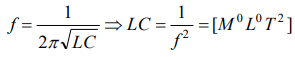## Units and Measurement Questions and Answers Part-15

1. Dimensional formula of heat energy is
a) $ML^{2}T^{-2}$
b) $MLT^{-1}$
c) $M^{0}L^{0}T^{-2}$
d) None of these

Explanation: Q = [ML2T-2] (All energies have same dimension)

2. If C and L denote capacitance and inductance respectively, then the dimensions of LC are
a) $M^{0}L^{0}T^{0}$
b) $M^{0}L^{0}T^{2}$
c) $M^{2}L^{0}T^{2}$
d) $MLT^{2}$

Explanation:3. The dimensions of "time constant"$\frac{L}{R}$ during growth and decay of current in all inductive circuit is same as that of
a) Constant
b) Resistance
c) Current
d) Time

Explanation:4. The period of a body under SHM i.e. presented by $T=P^{a}D^{b}S^{C}$   ; where P is pressure, D is density and S is surface tension. The value of a, b and c are
a) $-\frac{3}{2},\frac{1}{2},1$
b) $-1,-2,3$
c) $\frac{1}{2}-\frac{3}{2},-\frac{1}{2}$
d) $1,2,\frac{1}{3}$

Explanation:5. Which of the following pairs of physical quantities has the same dimensions
a) Work and power
b) Momentum and energy
c) Force and power
d) Work and energy

Explanation: Work and energy

6. The velocity of a freely falling body changes as $g^{p}h^{q}$  where g is acceleration due to gravity and h is the height. The values of p and q are
a) $1,\frac{1}{2}$
b) $\frac{1}{2},\frac{1}{2}$
c) $\frac{1}{2},1$
d) 1,1

Explanation:7. Which one of the following does not have the same dimensions
a) Work and energy
b) Angle and strain
c) Relative density and refractive index
d) Planck constant and energy

Explanation:8. Dimensions of frequency are
a) $M^{0}L^{-1}T^{0}$
b) $M^{0}L^{0}T^{-1}$
c) $M^{0}L^{0}T$
d) $MT^{-2}$

Explanation:9. Which one has the dimensions different from the remaining three
a) Power
b) Work
c) Torque
d) Energy10. The quantity $X=\frac{\epsilon_{0}LV}{t}:\epsilon_{0}$    is the permittivity of free space, L is length, V is potential difference and t is time. The dimensions of X are same as that of: